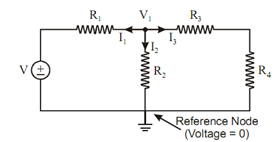## Application of Kirchhoffs Current Law Assignment Help

Assignment Help: >> Nodal and Mesh Analysis - Application of Kirchhoffs Current Law

Application of Kirchhoff's Current Law:

Kirchhoff's current law is utilized to determine the voltages at different nodes or junctions of the circuit. For this cause, Maxwell's node method is utilized. This method is being described with the help of an example given below.

Again consider the same circuit of Figure

For Applying Maxwell's Node Method

Suppose voltage V1 at node as shown in Figure and one reference node with zero voltage.Figure: Maxwell's Node Method

Assume three currents I1, I2 and I3 leaving the node V1, then apply KCL :

Algebraic of currents at any node is zero.

I1  + I 2  + I3  = 0

(V1 - V) /R1 + V1 /R2 + V1/ R3  + R4 = 0

V  [ 1/ R1 +  1 / R2 + 1/ (R3  + R4 )= V /R1

From this equation node, voltage V1 may be obtained and then current flowing through different elements may be determined.

For instance, current through R1 is

I  = (V1 - V) /R1

If value of I1 comes negative, it means actual direction of current I1 shall be opposite to the assumed direction as shown in Figure

Current division rule is also one of the applications of KCL.#### Assured A++ Grade

Get guaranteed satisfaction & time on delivery in every assignment order you paid with us! We ensure premium quality solution document along with free turntin report!

All rights reserved! Copyrights ©2019-2020 ExpertsMind IT Educational Pvt Ltd